# RD Sharma Solutions For Class 12 Maths Exercise 19.11 Chapter 19 Indefinite Integrals

RD Sharma Solutions for Class 12 Maths Exercise 19.11 Chapter 19 Indefinite Integrals is provided here. Evaluating the integrals of the form tan and secant functions along with non-negative integers by using binomial theorem is the main topic covered under this exercise. RD Sharma Solutions for Class 12 Maths is an essential tool for qualifying your exams with high marks.

It is created by our team of experts at BYJU’s, keeping in mind the latest CBSE norms and also in a simple language with suitable explanations. If you tend to study at the last minute, then this solution will help brush up all the important concepts and their formulae. To know more about this chapter, students can download RD Sharma Solutions for Class 12 Maths Chapter 19 Exercise 19.11 from the below-mentioned links.

## Download the PDF of RD Sharma Solutions For Class 12 Chapter 19 – Indefinite Integrals Exercise 19.11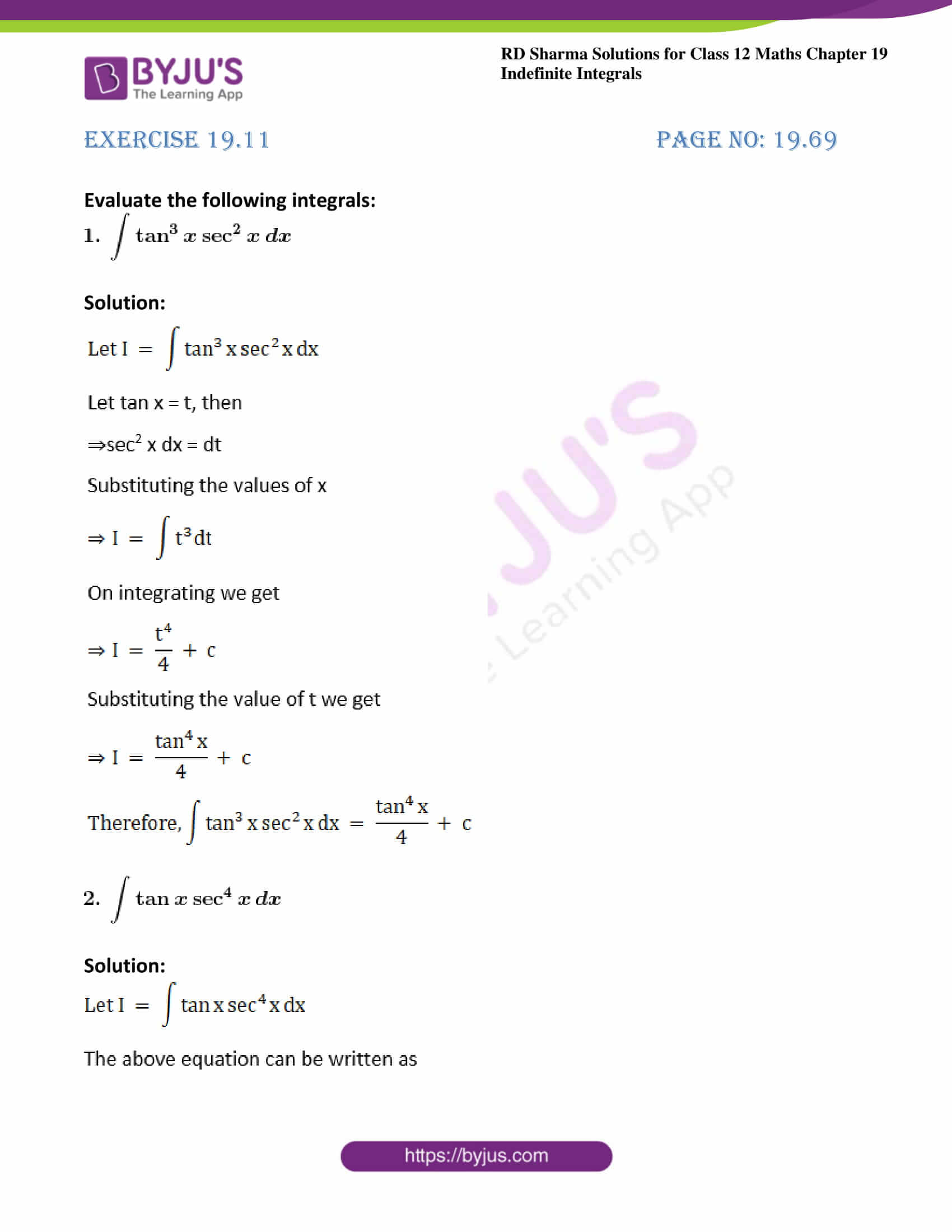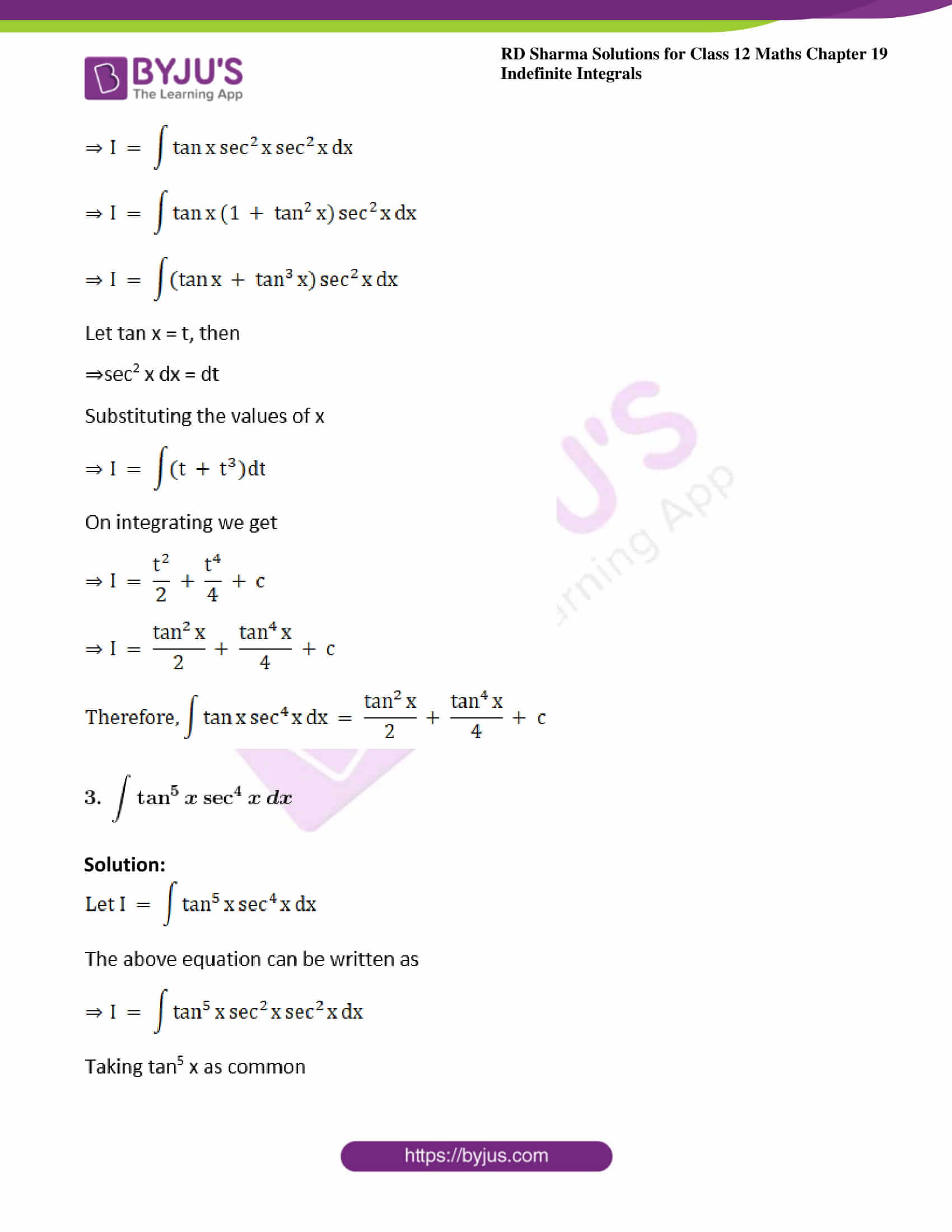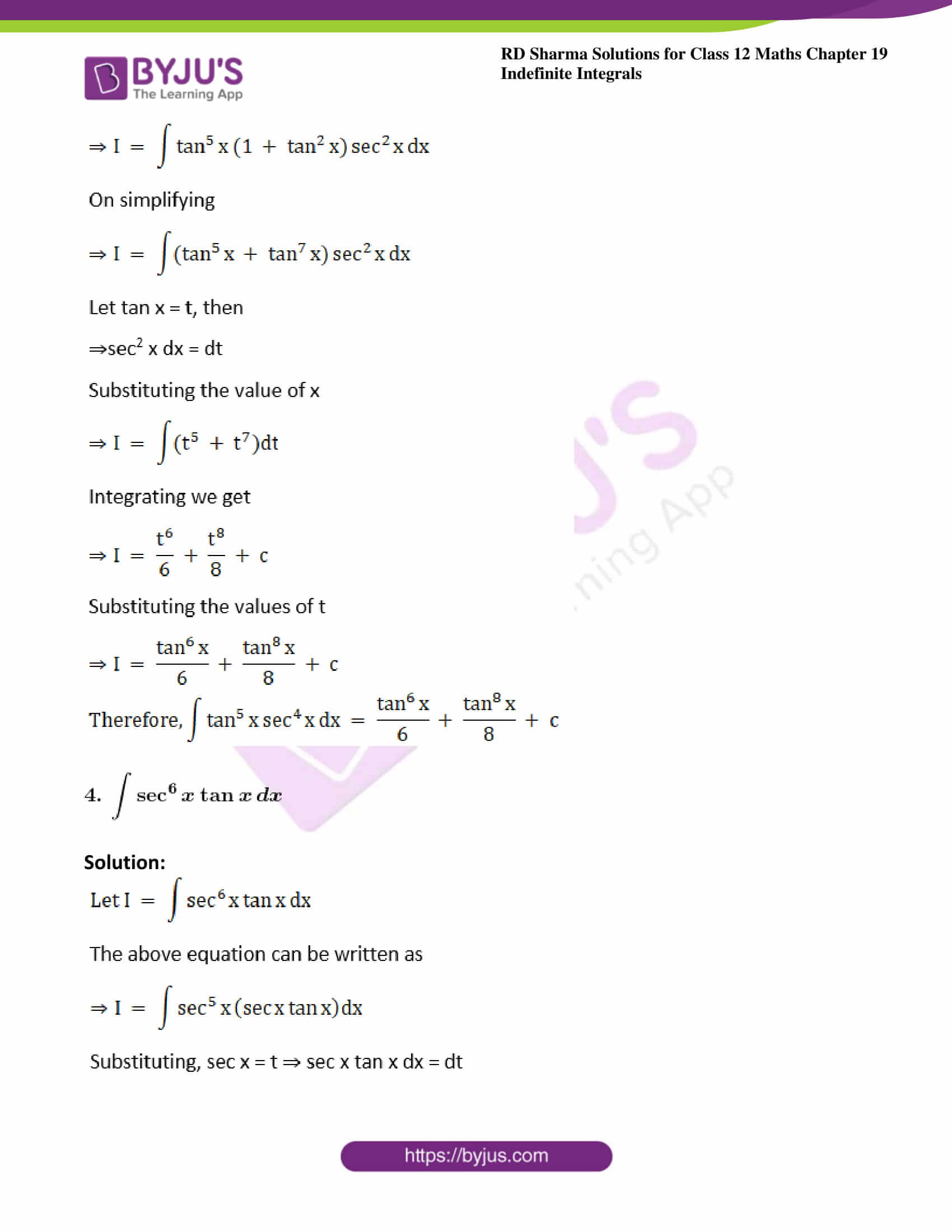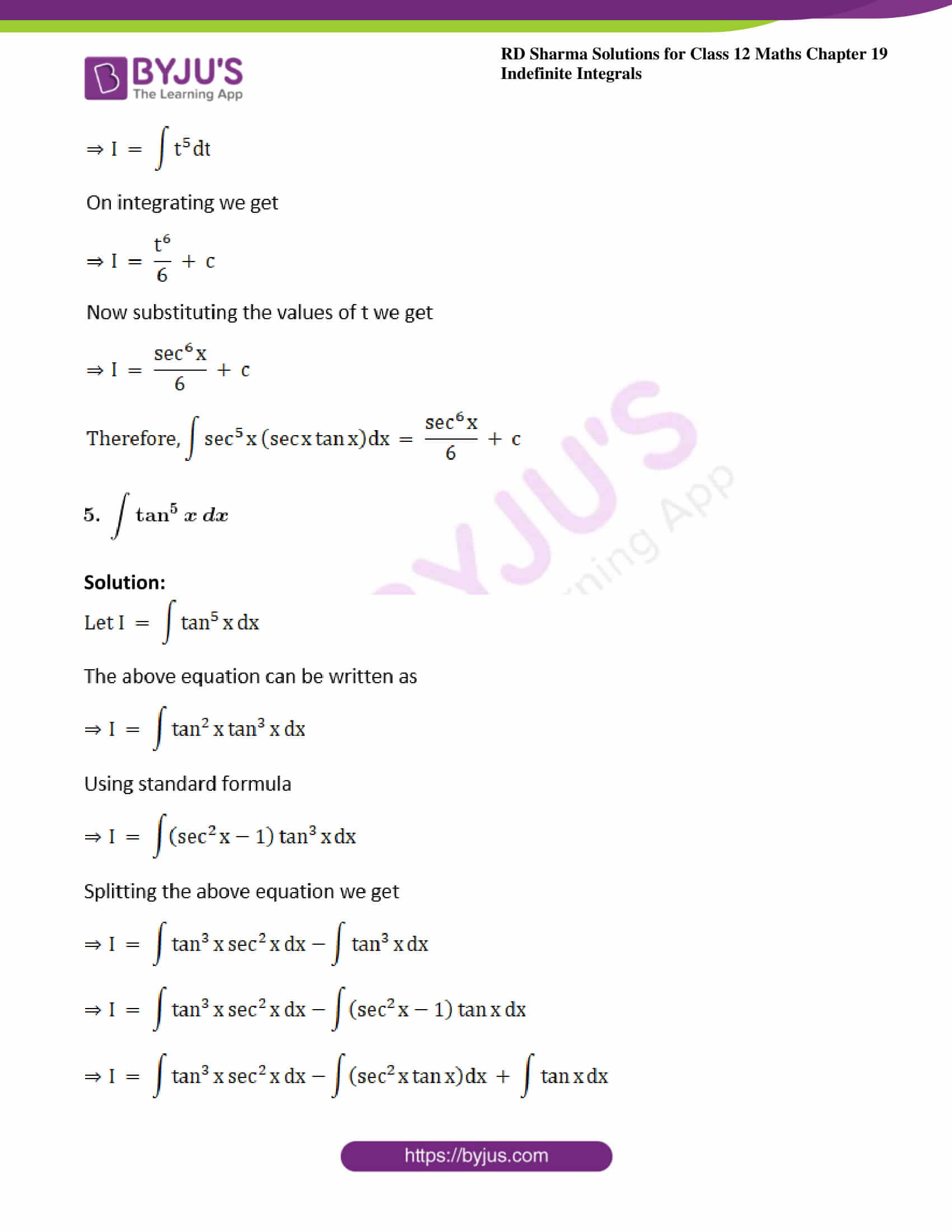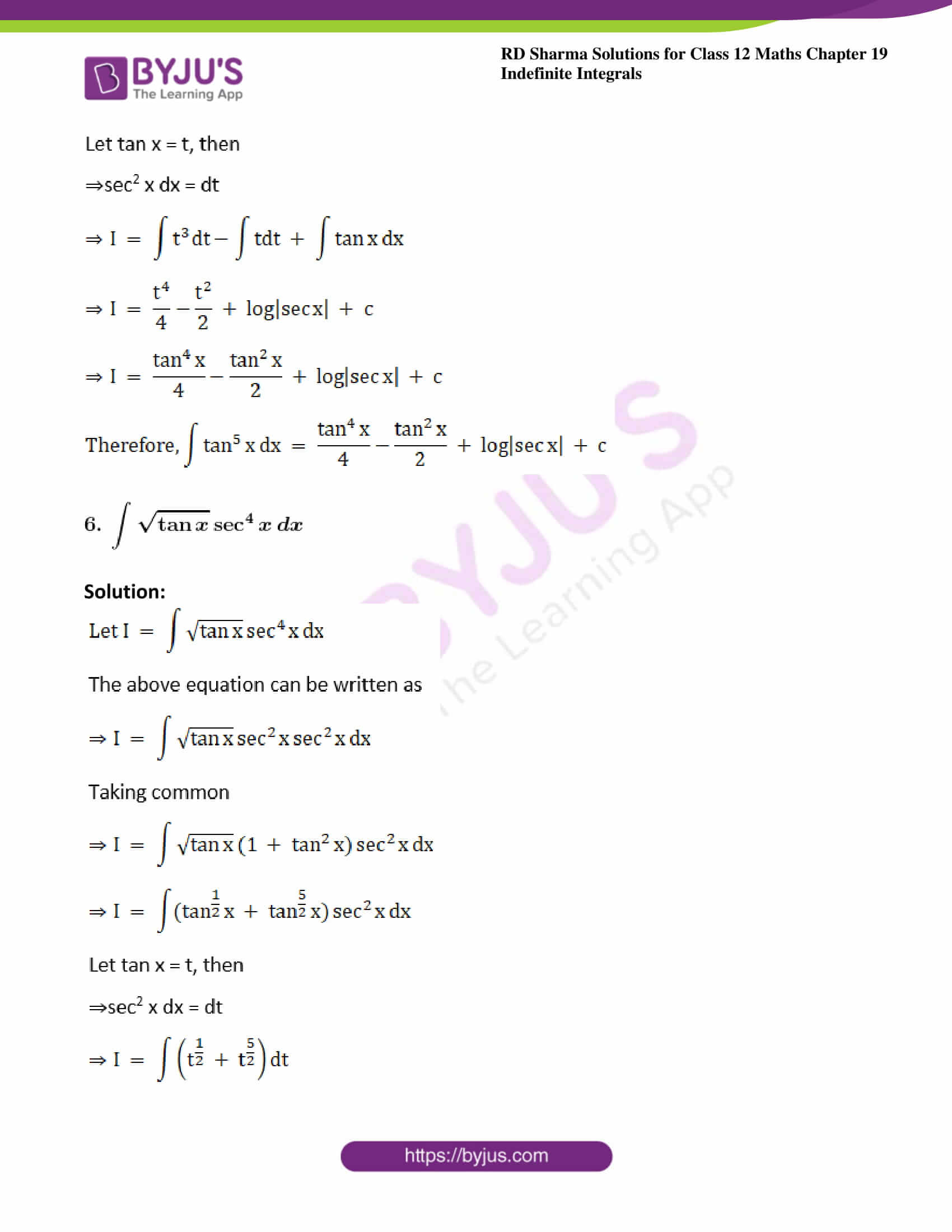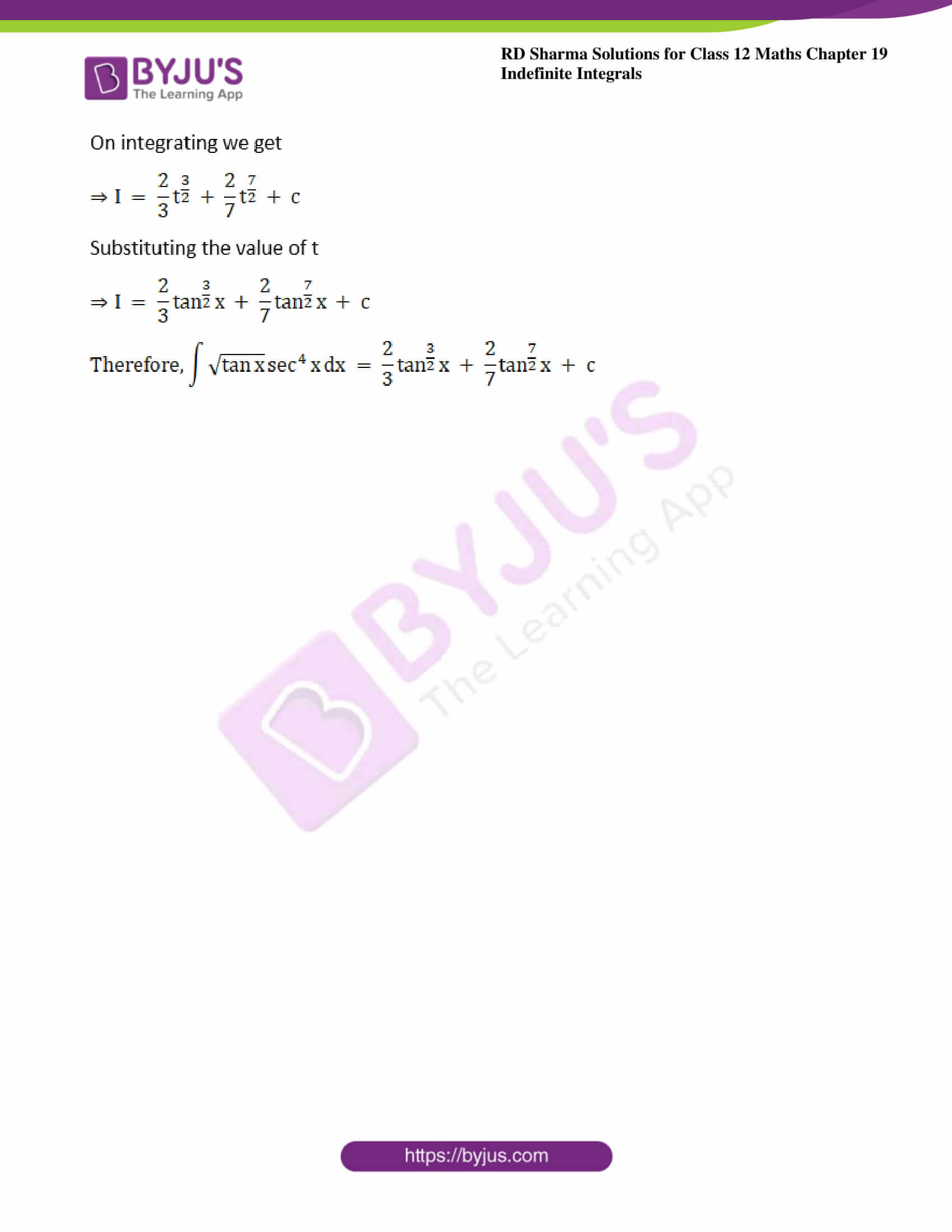### Exercise 19.11 Page No: 19.69

Evaluate the following integrals: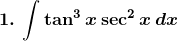Solution: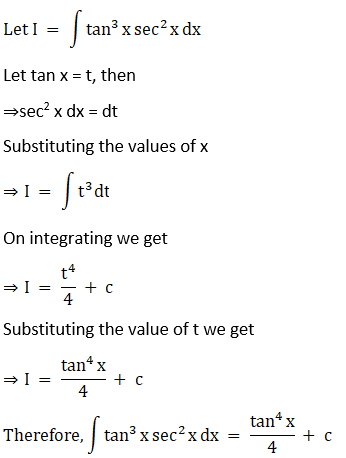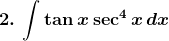Solution: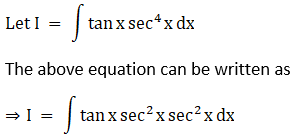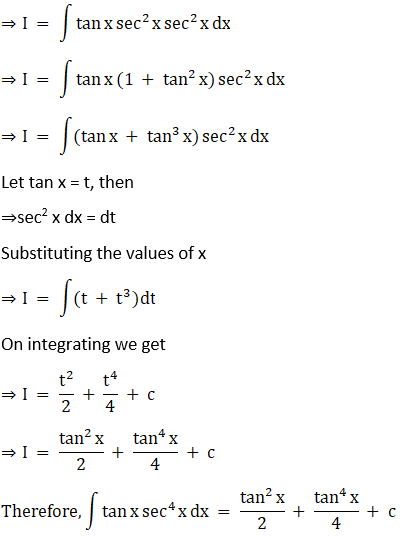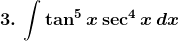Solution: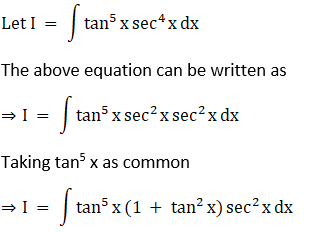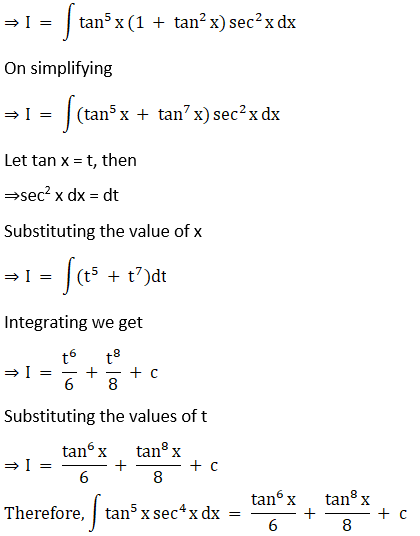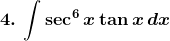Solution: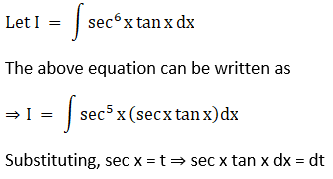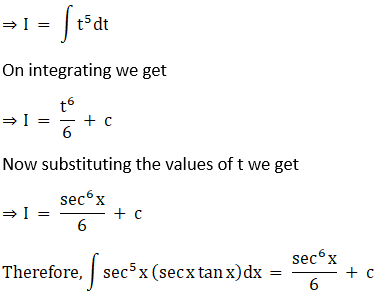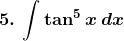Solution: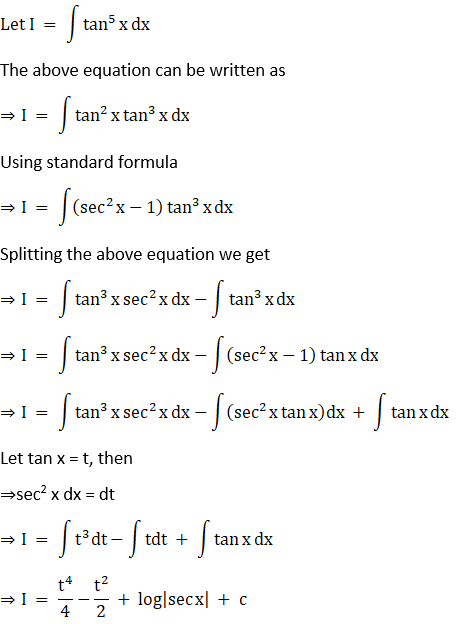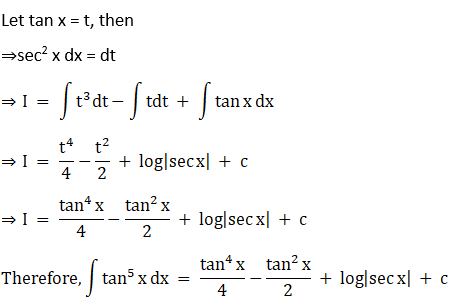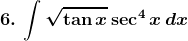Solution: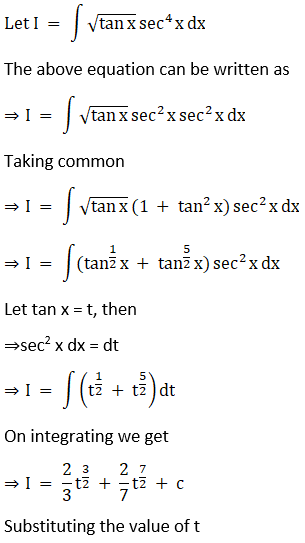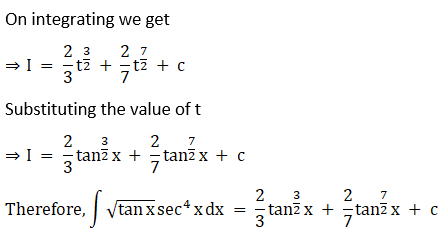### Access other exercises of RD Sharma Solutions For Class 12 Chapter 19 – Indefinite Integrals

Exercise 19.1 Solutions

Exercise 19.2 Solutions

Exercise 19.3 Solutions

Exercise 19.4 Solutions

Exercise 19.5 Solutions

Exercise 19.6 Solutions

Exercise 19.7 Solutions

Exercise 19.8 Solutions

Exercise 19.9 Solutions

Exercise 19.10 Solutions

Exercise 19.12 Solutions

Exercise 19.13 Solutions

Exercise 19.14 Solutions

Exercise 19.15 Solutions

Exercise 19.16 Solutions

Exercise 19.17 Solutions

Exercise 19.18 Solutions

Exercise 19.19 Solutions

Exercise 19.20 Solutions

Exercise 19.21 Solutions

Exercise 19.22 Solutions

Exercise 19.23 Solutions

Exercise 19.24 Solutions

Exercise 19.25 Solutions

Exercise 19.26 Solutions

Exercise 19.27 Solutions

Exercise 19.28 Solutions

Exercise 19.29 Solutions

Exercise 19.30 Solutions

Exercise 19.31 Solutions

Exercise 19.32 Solutions